# How to Find the Area of a Trapezoid and Rhombus

Hi, and welcome this video lesson on special types of quadrilaterals. Today we’re going be talking about trapezoids and rhombuses. Now if you’re looking for something on a normal quadrilateral, go ahead check our video out entitled common quadrilaterals.

For now, let’s get started on defining some of these objects. A trapezoid is a quadrilateral where at least 1 pair of sides are parallel. The parallel sides are called the bases, and the other 2 remaining sides are called legs.

Finding the area of one of these can be a bit trickier than other objects. The most common used equation is the following: Area is equal to the 2 bases, divided by 2, times H, or the height.

A rhombus is a little bit different. It’s a parallelogram where all 4 sides are of equal length and opposite angles are equal. Most often, it is referred to as a diamond.

To find the area of a rhombus, you can multiply the imaginary perpendicular lines that travel in the middle of a rhombus and divide the result by 2. Like this: L times M, divided by 2 is equal to the area. I hope that helps. Thanks for watching this video lesson and until next time, happy studying.

## Practice Questions

Question #1:

What is the area of this rhombus?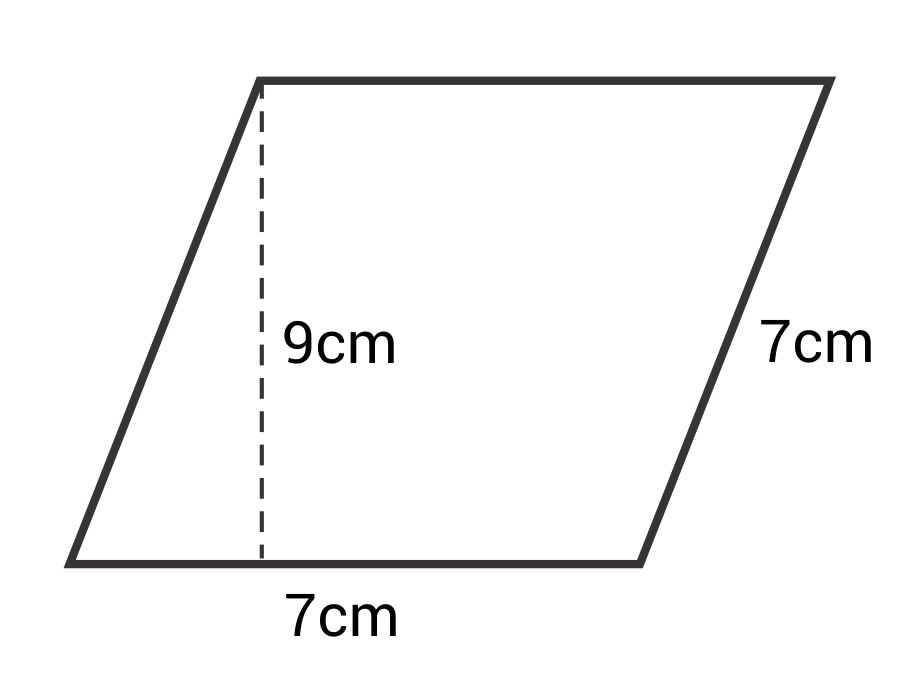28 cm2

31.5 cm2

14.5 cm2

63 cm2

The correct answer is 63 cm2. To find the area of a rhombus, use this formula:
$$A=bh$$
The base (b) is 7 cm and the height (h) is 9 cm.
$$A=(9)(7)=63\text{ cm}^2$$

Question #2:

What is the area of this trapezoid?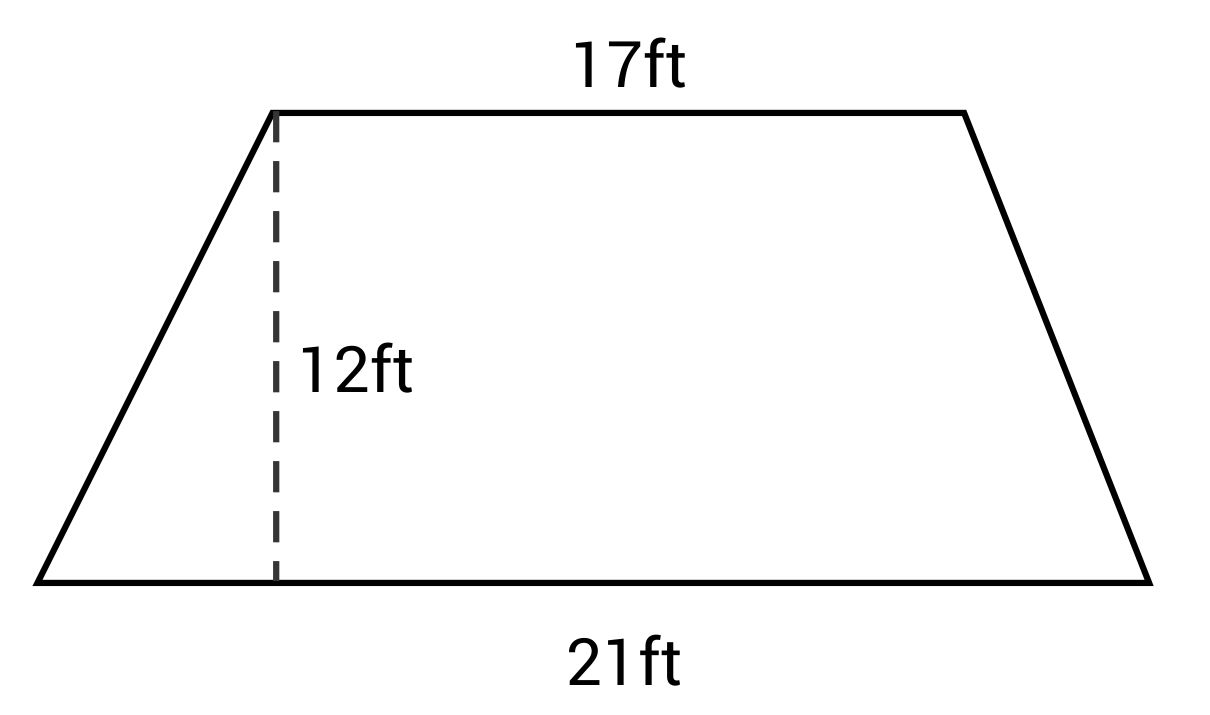147 ft2

228 ft2

375 ft2

456 ft2

The correct answer is 228 ft2. The formula for area of a trapezoid is:
$$A=\frac{1}{2}(b_1+b_2)h$$
The length of base 1 (b1) is 17 ft. The length of base 2 (b2) is 21 feet. And the height (h) is 12 ft.
$$A=\frac{1}{2}(17+21)(12)=\frac{1}{2}(38)(12)=228\text{ ft}^2$$

Question #3:

What is the area of this rhombus?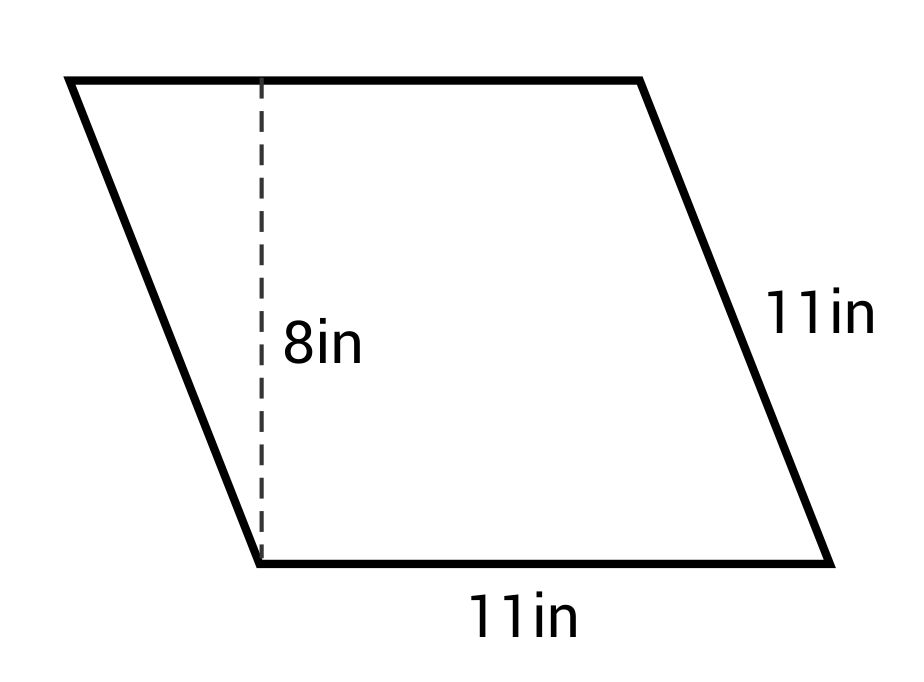88 in2

44 in2

38 in2

79 in2

The correct answer is 88 in2. The formula for area of a rhombus is:
$$A=bh$$
The base (b) is 11 in and the height (h) is 8 in.
$$A=(11)(8)=88\text{ in}^2$$

Question #4:

What is the area of this trapezoid?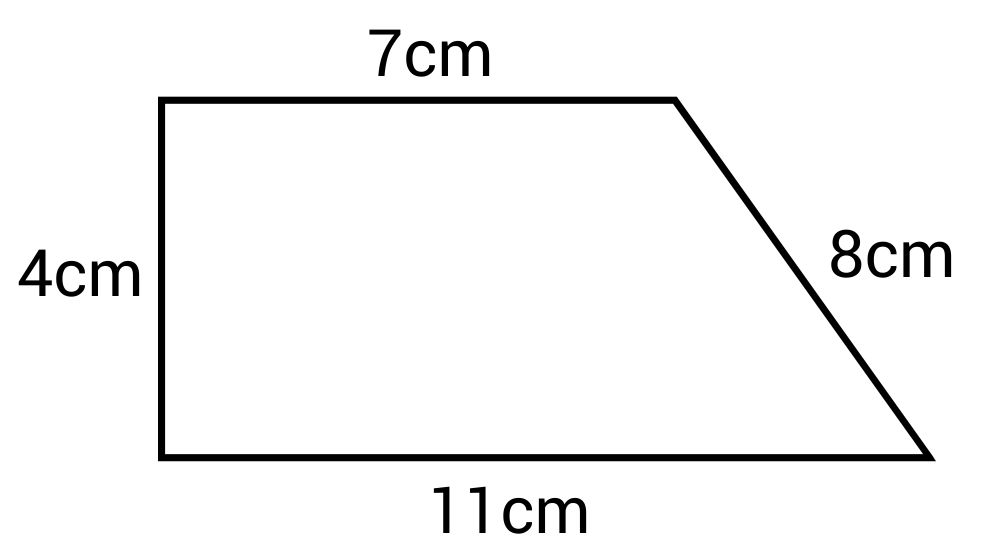39 cm2

30 cm2

25 cm2

36 cm2

The correct answer is 36 cm2. The formula for area of a trapezoid is:
$$A=\frac{1}{2}(b_1+b2)h$$
The length of base 1 (b1) is 7 cm. The length of base 2 (b2) is 11 cm. The length of the height (h) is 4 cm.
$$A=\frac{1}{2}(7+11)(4)=\frac{1}{2}(18)(4)=36\text{ cm}^2$$

Question #5:

What is the area of this rhombus?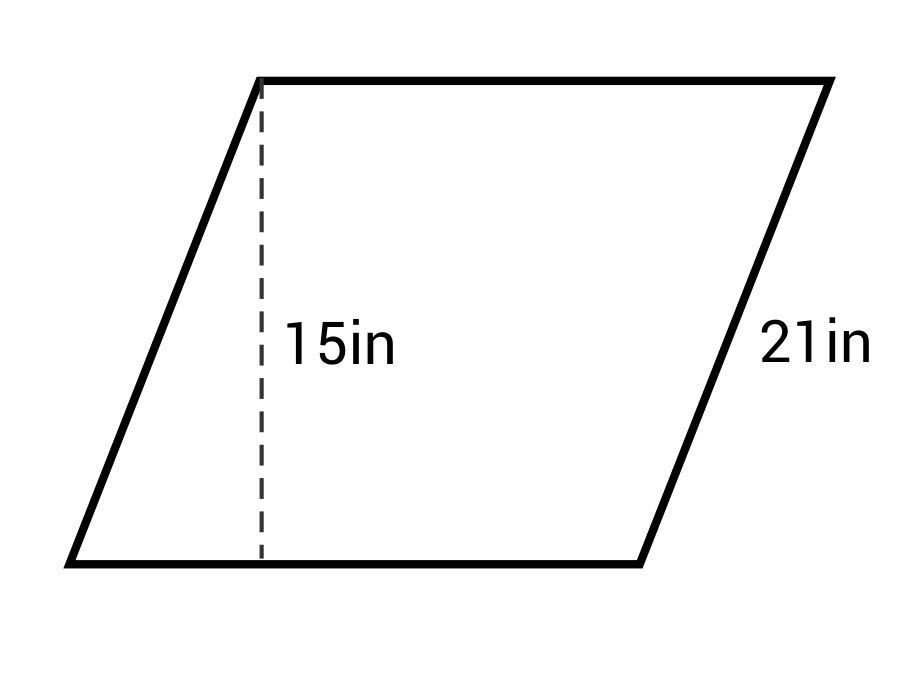157 in2

259 in2

315 in2

427 in2

The correct answer is 315 in2. The formula for area of a rhombus is:
$$A=bh$$
Since a rhombus has 4 congruent sides, the length of the base (b) is 21 in. The height is 15 in.
$$A=(21)(15)=315\text{ in}^2$$